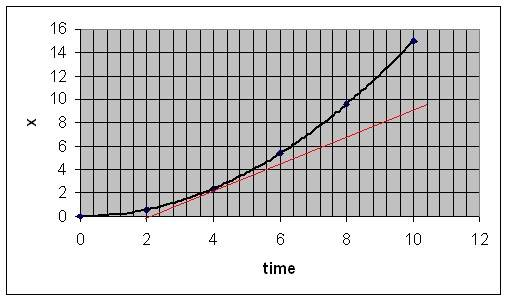Position-time graphs and tangent lines

The Following data table provides information about a crate of radishes that is sliding down the ramp of a delivery truck

time position
0 ------ 0
2------ 0.6
4-------2.4
6------- 5.4
8------- 9.6
10------ 15

Next it asks you to draw a position time graph, which i did, and its identical to the one in the curriculum. Then it asks you to draw 3 tangent lines, one at 4s, one at 6s, and one at 8s

Then it asks you to calculate the slopes of the tangents and put it in a time velocity table, which i have also done.

My question is why do we need a tangent line to find the slope? Cant we just use the simple slope formula to find the slope? v = d2-d2/t2-t2
Whats the point of a tangent slope? I dont get it.

Also this may seem trivial but can i use any 2 points on the line to find the slope? In this instance i used the given points along the line, for each tangent(4 seconds, 6 seconds, 8 seconds) i used the point, and the point before it to find the slope, i got the answers, and they were very close to the solution that the book has, and the book states that if the solutions are close then its good enough because some of the errors might be due to graphing and drawing of the tangent, even though i didnt use the graphical method to determine slope, i just use the formula. Because of this im thinking , oh since i used the slope formula, i shouldnt be off at all, but in my solution im off by like 0.2, all the answers however are very close, i got 0.9 m/s for 4 s, 1.5 m/s for 6s and 2.1 m/s for 8s.
Are these right? Sorry if it looks like i just put all my unorganized thoughts up here.

Delphi51
Homework Helper
Yes, your calcs with the points should be close. When you use the points at 4 and 6 seconds, the slope you find will be equal to the slope of the curve itself at some time between 4 and 6. You don't know what that time is exactly, so you won't have a definite time to put in your velocity/time table. You could guess 5 seconds, but you don't really know.

If you draw a smooth curve through the x,t points and a tangent line accurately (and that is an art as well as a skill), you are getting the slope at a known time - where the line touches the curve.

I have a pretty experienced eye, and I think your slope at time 4 is a little too low.Last edited:
#edit, someone said that v=d2-d1/t2-t1 is not for slope but for avg velocity, but since in this game we are dealing with position time graph, the velocity is the slope, am i right?

Delphi51
Homework Helper
Your slope of the secant line through two points is an average velocity over the interval t1 to t2. The instantaneous velocity at time t is the slope of the tangent line that touches the curve at t.